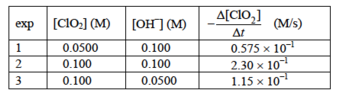# Problem: A kinetic study of the reaction 2 ClO2(aq) + 2 OH -(aq) → ClO2- (aq) + ClO3- (aq) +   H2O(l) yielded the data shown on the image. A.  What is the rate of appearance of the ClO3- in experiment 1 (Be sure to inlcude units in this and other answers.)   B. Determine the experimental rate law.   C. Determine the value of the rate constant  based on your answer to Part C. (Inlude units)

###### FREE Expert Solution
90% (299 ratings)
###### Problem Details

A kinetic study of the reaction

2 ClO2(aq) + 2 OH -(aq) → ClO2- (aq) + ClO3- (aq) +   H2O(l)

yielded the data shown on the image.

A.  What is the rate of appearance of the ClO3- in experiment 1 (Be sure to inlcude units in this and other answers.)

B. Determine the experimental rate law.

C. Determine the value of the rate constant  based on your answer to Part C. (Inlude units)What scientific concept do you need to know in order to solve this problem?

Our tutors have indicated that to solve this problem you will need to apply the Rate Law concept. You can view video lessons to learn Rate Law. Or if you need more Rate Law practice, you can also practice Rate Law practice problems.

What is the difficulty of this problem?

Our tutors rated the difficulty ofA kinetic study of the reaction 2 ClO2(aq) + 2 OH -(aq) → C...as high difficulty.

How long does this problem take to solve?

Our expert Chemistry tutor, Jules took 5 minutes and 59 seconds to solve this problem. You can follow their steps in the video explanation above.

What professor is this problem relevant for?

Based on our data, we think this problem is relevant for Professor Castaneda's class at UMICH.